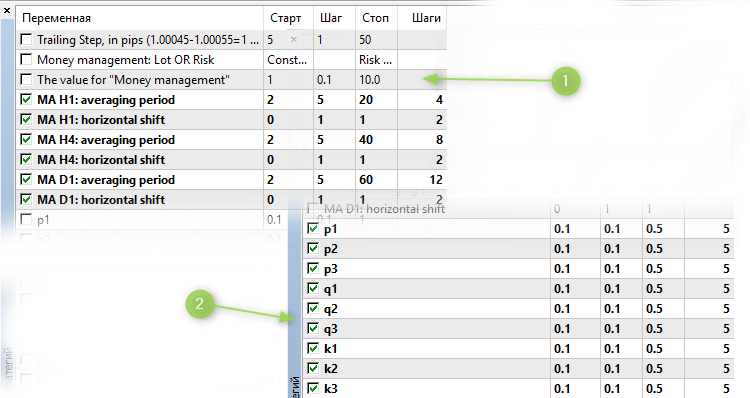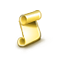Interesting script?
So post a link to it -
let others appraise it
You liked the script? Try it in the MetaTrader 5 terminal# Three neural networks - expert for MetaTrader 5

Views:
10431
Rating:
Published:
2019.02.07 09:09

Idea by: Apelsin

mq5 code by: barabashkakvn

The EA uses a very simple neural network unit

```   double n1=((ma_h1-ma_h1)/ma_h1)*p1+((ma_h1-ma_h1)/ma_h1)*p2+((ma_h1-ma_h1)/ma_h1)*p3;
n1=(MathRound(n1*10000));

double n2=((ma_h4-ma_h4)/ma_h4)*q1+((ma_h4-ma_h4)/ma_h4)*q2+((ma_h4-ma_h4)/ma_h4)*q3;
n2=(MathRound(n2*10000));

double n3=((ma_d1-ma_d1)/ma_d1)*k1+((ma_d1-ma_d1)/ma_d1)*k2+((ma_d1-ma_d1)/ma_d1)*k3;
n3=(MathRound(n3*10000));

if((n1>0 && n2>0 && n3>0))
if((n1>0 && n2<0 && n3<0))
m_need_open_sell=true;
```

based on the signals from three indicators iMA (Moving Average, MA) on H1, H4, and D1.

Recommendations on Optimization

Optimization is performed in two stages (1 and 2 in the picture below).

After stages one and twoselect among the top ten ones the version that provides equally good results on both backtest and forward.

Translated from Russian by MetaQuotes Software Corp.
Original code: https://www.mql5.com/ru/code/23338Daily range

Searching for a daily range. We use OBJ_HLINE, OBJ_VLINE.SetColorFiboLevels_Grand_xN

The script builds the Fibonacci Levels graphical object with the option of selecting the number of its segmentsSupremAutoFibo

The indicator builds graphical object Fibonacci Levels with many settings at High and Low prices over the period specified in the indicator input parametersExp_SpearmanRankCorrelation_Histogram_TimeWeekPeriod

Expert Advisor Exp_SpearmanRankCorrelation_Histogram with the added option of trading within the weekly range only, the borders of which are specified in the EA's input settings